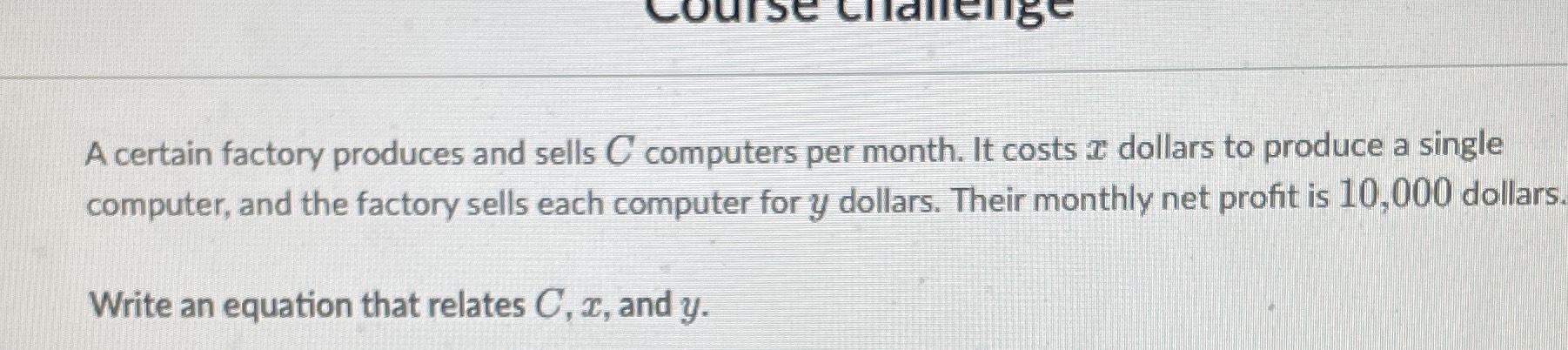### ¿Todavía tienes preguntas de matemáticas?

Pregunte a nuestros tutores expertos
Algebra
PreguntaA certain factory produces and sells $$C$$ computers per month. It costs $$x$$ dollars to produce a single computer, and the factory sells each computer for $$y$$ dollars. Their monthly net profit is $$10,000$$ dollars. Write an equation that relates $$C , x ,$$ and $$y$$ .